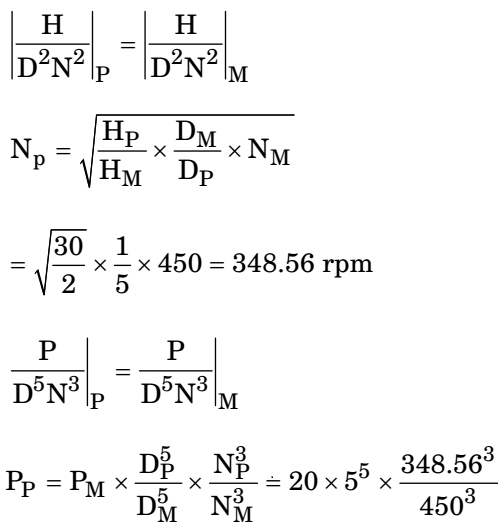## Fluid Mechanics and Hydraulic Machinery Miscellaneous

#### Fluid Mechanics and Hydraulic Machinery

1. The SI unit of kinematic viscosity v is

1. Kinematic Viscosity:

 v = Dynamic viscosity = μ Density ρ

 v = μ = Ns / m² ρ kg / m3

 = kg.m × s × 1 s² m² kg / m3

= m²/s

##### Correct Option: A

Kinematic Viscosity:

 v = Dynamic viscosity = μ Density ρ

 v = μ = Ns / m² ρ kg / m3

 = kg.m × s × 1 s² m² kg / m3

= m²/s

1. Match List-I with List-II and select the correct answer using the codes given below the lists:
List-I
P. Centrifugal compressor
Q. Centrifugal pump
R. Pelton wheel
S. Kaplan turbine
List-II
1. Axial flow
2. Surging
3. Priming
4. Pure impulse enter codes:

1. Kaplan – Axial flow Pelton – Impulse type

##### Correct Option: A

Kaplan – Axial flow Pelton – Impulse type

1. A phenomenon is modeled using-n dimensional variables with k primary dimensions. The number of non-dimensional variables is

1. NA

##### Correct Option: C

NA

1. The parameters which determines the friction factor for turbulent flow in a rough pipe are

1. NA

##### Correct Option: C

NA

1. A test is conducted on a one-fifth scale model of a Francis turbine under a head of 2 m and volumetric flow rate of 1 m3/s at 450 rpm. Take the water density and the acceleration due to gravity as 103 kg/m3 and 10 m/s2, respectively. Assume no losses both in model and prototype turbines. The power (in MW) of a full sized turbine while working under a head of 30 m is _______ (correct to two decimal places).

1.  Dm = 1 Dp 5

Model: H = 2 m, Q = 1 m3 /s
Prototype: H = 30 m, P =?
N = 450 rpm, ρ = 1000 kg/m3 /g = 10 m/s2
For model, pm = ρgQH = 1000 × 10 × 1 × 2 = 20 kW= 29045.24 kW
= 29.05 mW

##### Correct Option: C

 Dm = 1 Dp 5

Model: H = 2 m, Q = 1 m3 /s
Prototype: H = 30 m, P =?
N = 450 rpm, ρ = 1000 kg/m3 /g = 10 m/s2
For model, pm = ρgQH = 1000 × 10 × 1 × 2 = 20 kW= 29045.24 kW
= 29.05 mW